# Complex numbers

Complex numbers are mainly used in electrical engineering. Just think of the phase shift of inductive and capacitive loads or the calculation of complex resistances. So let´s take the first steps together to understand these complex numbers.

## Overview -Number sets in mathematics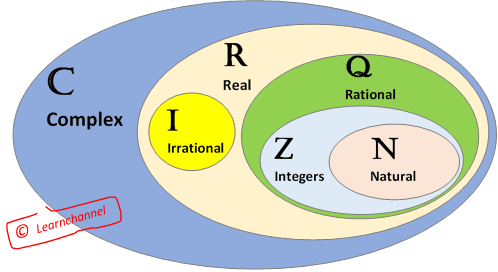Number sets in mathematics

The numbers you can make by dividing one integer by another are named as Rational Numbers Q (Q stands for "quotient"). In other words, fractions.

Examples: 5/2 (= 2.5), 10/5 (= 2), -3/100 (= -0.3)

An Irrational Number is a real number that cannot be written as a simple fraction.

Example:  π= 3,1415…, Euler`s number e = 2,71828... , √2 = 1,41421...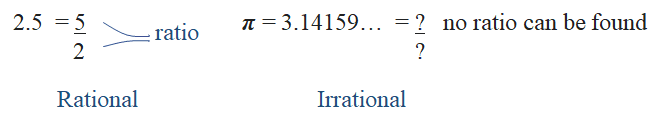Difference between rational and irrational number

Natural Numbers can mean either "Counting Numbers" {1, 2, 3, ...}, or "Whole Numbers" {0, 1, 2, 3, ...}, depending on the subject.

Integers are like whole numbers, but they also include the negative numbers. Zero is also included.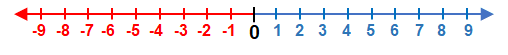Definition Integer

A complex number exist of a real part and an imaginary part: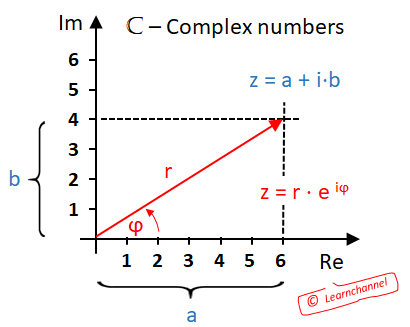Complex numbers - Complex Plane

A complex number exist of a real part and an imaginary part. You can describe a complex number as: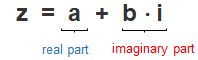Defing a Complex number

As you can see, all real numbers are also complex numbers with an imaginary part of zero.

This type of representation is referred to as algebraic form. Another possibility of representation is the trigonometric form and derived from it the Eulers formula. This is where the pointer length and angle are specified:

z = r × e

... soon coming more